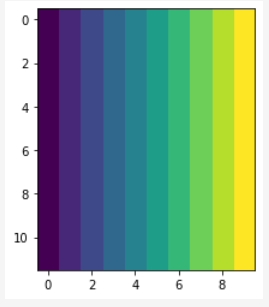# Grid sample and align corners

In the code below, I’ve created a simple image:and then applied grid_sample to it using scaled integer row/col coordinates. The goal is to reproduce the input image exactly, and I achieve this using `align_corners=True`. However, I can’t think geometrically how to set up the same problem to work with `align_corners=False`. I want to understand this, as I prefer to leave default values as is in practice.

Here’s my example:

``````image = torch.arange(0., 10., requires_grad=True).expand(12, 10)
image = image[None, None]

row_coords = torch.linspace(0, 11, 12)
col_coords = torch.linspace(0, 9, 10)
row_col_coords = torch.cartesian_prod(row_coords, col_coords)

image_dims = torch.tensor(image.shape)[-2:]
ratio_spanned = (row_col_coords) / (image_dims - 1)
scaled = ratio_spanned * 2
normalized_coords = -1 + scaled

normalized_coords = normalized_coords.flip(dims=(-1,))
normalized_coords = normalized_coords[None, :, None, :]

intensities = F.grid_sample(input=image, grid=normalized_coords, align_corners=True)
plt.imshow(intensities.view(12, 10).squeeze().detach())
``````But with `align_corners=False`:What is the correct way to set up the dimensions and scaling such that default values work?